Email Us: info@lupinepublishers.comCall Us: +1 (914) 407-6109   57 West 57th Street, 3rd floor, New York - NY 10019, USA

# Lupine Publishers Group

## Lupine PublishersISSN: 2641-6794# A Mechanics-Based Procedure for Predicting Groundwater-Rise-Induced Slope Displacements Volume 1 - Issue 4

Ching-Chun Huang*

• Professor, Department of Civil Engineering, Taiwan

Received: November 12, 2018;   Published: November 27, 2018

Corresponding author: Ching-Chun Huang, Professor of Department of Civil Engineering, National Cheng Kung University, Taiwan, Email: samhcc@mail.ncku.edu.tw

DOI: 10.32474/OAJESS.2018.01.000117

## Abstract

A procedure for predicting cumulative slope displacements induced by groundwater table changes is proposed. The proposed procedure incorporates a back-calculation technique for the soil strength parameter based on the records of slope displacement and ground water table changes. The analytical tool for the back-calculation is a limit-equilibrium-based finite displacement method (FFDM) requiring force and moment equilibrium, displacement compatibility, and a hyperbolic shear stress-displacement constitutive law. The analyzed potential sliding mass is a statically determinate system, providing a closed-form solution for the displacement of the slope. Two well documented case histories of periodic rainfall-induced slope displacements are used to validate the proposed analytical procedures. The hyperbolic soil strength parameters back-calculated from the first event of slope displacement can be used as operational soil strength parameters for predicting subsequent slope displacements caused by rainfall-induced groundwater table elevation changes. The proposed method alleviates possible difficulties associated with the evaluation of soil strength using undisturbed soil sampling of colluviums in foothill areas. The proposed method requires little computer time in deriving useful information of slope displacement which cannot be achieved using conventional limit equilibrium methods or advanced numerical analyses. The proposed analytical procedure is valid only for simulating instantaneous sliding where time-dependent viscous (or creep) deformation is not dominant.

## Introduction

A substantial part of the infrastructure damage and remediation budget is used to fix damage caused by slope failures [1,2]. Therefore, analyses for slope failure potential in response to environmental changes, such as intensive rainfall, seismic activities  or groundwater level changes  is necessary for facilitating maintenance and disaster mitigation programs. Various analytical methods have been developed for slope stability evaluations. In the study of important and large-scale infrastructure such as dams or metropolitan shield tunnels where failures are lifethreatening, sophisticated numerical methods, such as the finite element method (FEM) and the finite difference method (FDM), are desirable . In such cases, sufficient budgets for intensive ground explorations, computer analysis, and skillful computational engineers are needed. In reality, studies on slope stability are frequently time- and budget-limited, and a numerically accurate (or sophisticated) computer analysis often makes numerous assumptions regarding the underground geological or geo environmental conditions. A complete numerical analysis with totally known material properties and underground environmental conditions is rare. This may explain why limit equilibrium methods, in which the displacement (or strain) is not an analytical outcome, are still popular among geotechnical engineers in studying slope engineering problems. Most conventional limit equilibrium slope stability methods are based on the principle of limit equilibrium (or force equilibrium), providing a single value of safety factor (Fs) for the analyzed slope without considering displacement-related information for the potential sliding soil mass. A time-efficient tool capable of calculating slope displacements is necessary. A forceequilibrium- based finite displacement method (FFDM) proposed by Huang  is used here.

## methodology

### Object of Study

The method of FFDM proposed by Huang  and extended by Huang , Huang and Yeh  and Huang  constitutes the core of back-calculations and predictions discussed in the following. It has been previously shown that for a sliced potential failure mass confined by a slip surface with an arbitrary shape, as shown in Figure 1, the system is a statically determinate one. A constitutive law for the shear stress vs. shear displacement relationships based on the hyperbolic soil model (parameters: K, n, and Rf) for the soil constituting the slip surface is incorporated. In addition, the Mohr- Coulomb failure criterion (parameters: cohesion, c and internal friction angle, Ф), and local safety factor, F Si, the displacement compatibility between adjacent slices is considered by using displacement diagrams (displacement compatibility function f(αi)). The vertical displacement at the top of the potential sliding mass (D0) and shear displacement along the sliding surface (D1- Dns; ns: total number of slices). Figure 2 shows the flow chart of the computer program SLICE-DISP for the proposed FFDM.

### Case History: Woo-Wan-Chai Slope

Figure 4: Records of long-term monitoring for case history #2: (a) Daily rainfall; (b) Groundwater table heightsFigure 5: Conventional safety factors in response to the groundwater table fluctuations for case history #1 (Woo-Wan-Chai).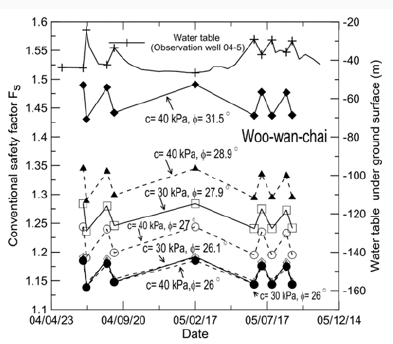Figure 3 shows a cross section of the Woo-Wan-Chai slope [9,10] consisting of alluviums. The slope is a part of a highway winding through the western foothills of Taiwan. The slope is subjected to rainfall-induced periodic displacements, requiring repeated remedial measures. Figures 4a & 4b show records of daily rainfall and groundwater table heights, respectively, during the period of May 2004 to October 2005. The majority of high daily rainfall is caused by typhoons. A close relationship between intensive rainfalls and rises of the groundwater table can be seen. The slip surface presented in Figure 3 is formed by connecting points of abrupt deflection detected by the inclinometers available in-situ. The slip surface reaches a maximum depth of about 90 m below slope surface. According to the values of Rock Quality Designation (RQD) as shown in Figure 3, the weather rock strata have scattered RQD values, occasionally showing a low RQD= 10%, suggesting the investigated slip surface is associated very weak soil-like material. Due to the difficulty associated with undisturbed sampling in weathered rock stratum, soil shear strength can only be estimated indirectly from the values of RQD. Values of internal friction angles (ϕ) ranging between 260c-350c are assumed in the following analyses. The first event of heavy rainfall (or groundwater table rise), caused by typhoon Mindulle on July 1, 2004, is used for calibrating in-situ soil parameters K, n, and Rf. The measured groundwater tables before and after rainfall shown in Figure 3 are used in the calibration of the in-situ soil parameters. Figure 5 shows the safety factors of the slope (Fs) calculated using the Janbu’s slice method with various input values of c and φ. It can be seen that during the period of monitoring, values of Fs varied to some extent according to the level of the groundwater table. However, these values of Fs suggest that the slope is stable and thus do not reflect the measured slope displacement.

Figure 6: Results of parametric studies on case history #1 (Woo-wan-chai) using wide ranges of K, n, c and (a) Rf=0.7; (b) Rf=0.8; (c) Rf=0.9.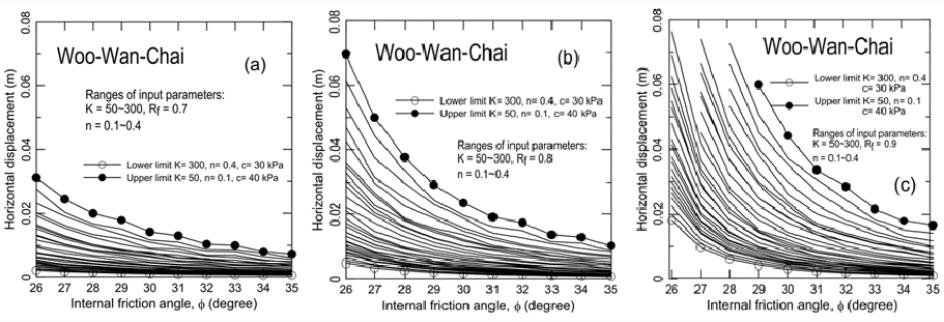Figure 7: Records of long-term monitoring of case history #2: (a) Daily rainfall; (2) Groundwater table heights.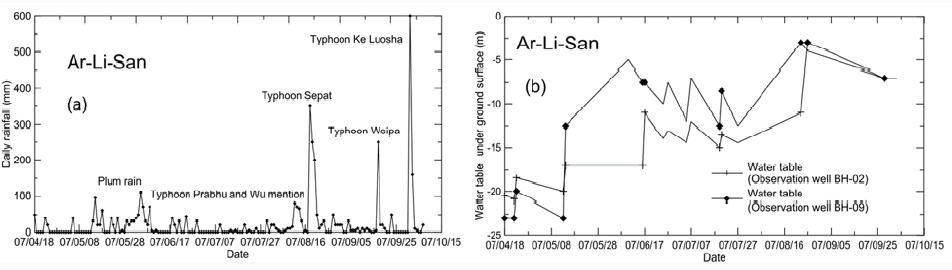Figures 6(a-c) show the calculated horizontal displacement at the location of inclinometer 04-4 (which is 280.5 m from the crest of the slip surface) for the first rainfall event using Rf= 0.7, 0.8, and 0.9, respectively. In Figures 6(a-c), values of K= 50, 100, 200, 300; n= 0.1, 0.2, 0.3, and 0.4; φ=260c-350c were used to account for possible ranges of soil parameters. The reasons for using these ranges of Rf, K, and n are given in the Discussion section. For all cases, c= 0 was applied to the entire zone of the slope mass for the post-rainfall condition; c= 30 kPa was applied to the above-groundwater-table zone for the pre-rainfall condition. To obtain the calibrated values of K, n, Rf, and φ, horizontal lines were drawn at 30 mm (measured horizontal displacement from inclinometer 04-4 for the first event of slope movement) in Figures 7(a-c). Values of K and n that represent the upper bound, the intermediate value, and the lower bound of ϕ that generate a slope displacement of 30 mm were selected as the back-analyzed values of soil parameters. A total of seven sets of K, n, and Rf, are used for evaluating the slope displacements induced by the subsequent events of rainfall (or groundwater table rises). Figure 8 shows a comparison of calculated and measured cumulative slope displacements at the location of 280.5 m from the crest of the slip surface (at inclinometer 04-4). The calculation of cumulative slope displacements assumed that a plastic (or irreversible) slope displacement occurs for each rainfall event. The cumulative slope displacement measured at 280.5 m for the crest of the slip surface is also shown. A good agreement between the measured and calculated slope displacements (with a maximum error of 10%) can be seen. The fact that seven sets of input K, n, and Rf all generate similar values of cumulative slope displacement reveals that the final results of calculation are insensitive to the input parameters provided that the values of K, n and Rf, and φ are calibrated using the first event of slope movement

Figure 8: Comparison of measured and calculated long-term slope displacements for case history #1.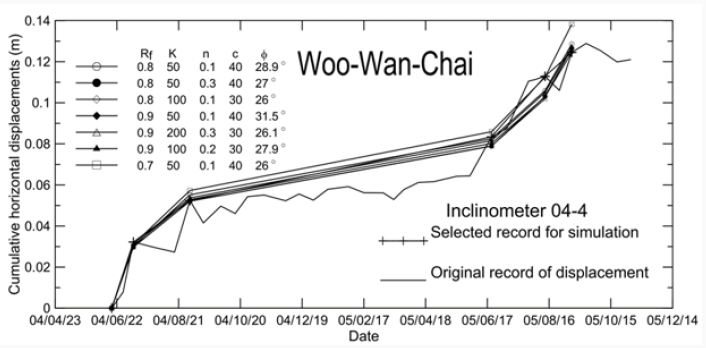### Case History: Ar-Li-San Slope

Figure 9 shows the cross section of the Ar-Li-San slope which is on the same highway as the slope in case history no. 1 but at a different location. A maximum depth of slip surface of about 25 m has been detected by the inclinometers shown in Fig. 8. The borehole data shown in Fig. 8 suggest that the slip surface locates in highly weathered rock strata which frequently show RQD values as low as zero. Therefore, a soil-like material is likely to prevail along the slip surface, and φ= 250c -360c is assumed in the following analyses. Figures 7 (a & b) show the daily rainfall and groundwater table levels, respectively, recorded during April-August 2007. Figure 10 shows the changes of safety factors associated with the fluctuation of the groundwater table. Figure 10 reveals a generally descending trend of safety factors in response to the generally ascending groundwater table during the rainy season. However, information on the performance of the slope during the rainy season is also lacking, as discussed for Figure 5.

Figure 10: Sefety factors calculated using Janbu’s slice method during the period of monitoring.Figure 11: Parametric studies for the slope displacements using various input parameters: (a) Rf=0.7; (b) Rf=0.8; (c) Rf=0.9.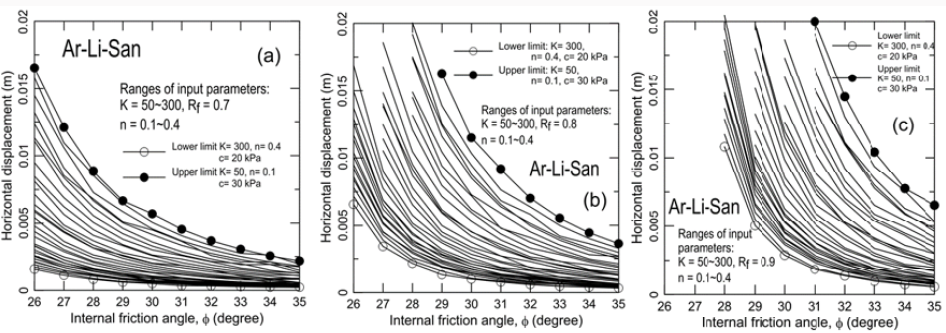Figure 12: Comparisons of measured and calculated cumulative slope displacements for case history #2.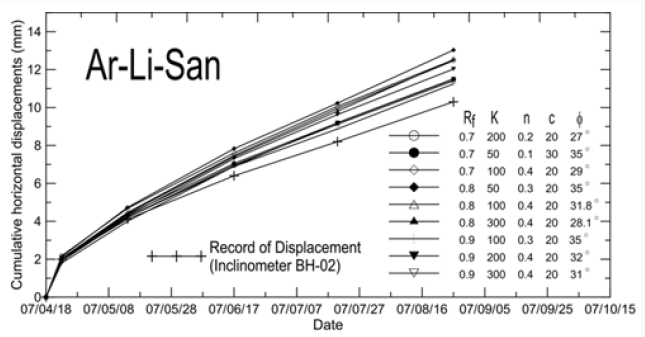Figures 11(a-c) show horizontal displacements at the location of 113 m from the crest of slip surface, calculated using Rf= 0.7, 0.8, and 0.9, respectively. Similar to those used for case history #1, K= 50, 100, 200, and 300; n=0.1, 0.2, 0.3, and 0.4; and φ=260c- 350c were used. A total of nine sets of K’s, n, and Rf were back-calculated based on an approach similar to that used in case history no. 1 for a measured horizontal slope displacement of 3 mm from the inclinometer (BH-02) in case history no. 2. Figure 12 shows comparisons between the measured and calculated cumulative slope displacements. The trends of all calculated curves of cumulative slope displacement are close to that of slope displacement during the rainy season. It can be seen that for the five simulated events, the percentage error ranges between 10% and 20%, which is larger than that for case history no. 1 partially due to the fact that the slope displacement in the monitoring season was relatively small (a few mm). A 20% error for slope displacement prediction is considered acceptable. This result confirms that the calculated cumulative slope displacement is insensitive to the input parameters provided that they are back-calculated from the first event of slope movement.

## Conclusion

A mechanics-based procedure for predicting continuous slope displacements induced by periodic groundwater table changes was proposed. A limit-equilibrium-based finite displacement method (FFDM) previously proposed by the author was used to calibrate soil parameters based on two long-term records of periodic slope displacements induced by some intensive rainfall (or groundwater table fluctuations). The proposed approach consists of a calibration procedure that is used to find possible representative soil strength and displacement-related parameters based on the first event of slope movement induced by a groundwater table rise. These backcalculated soil parameters were then used to predict subsequent slope displacements in response to the groundwater table rise induced by intensive rainfall. Two case histories were used to verify the proposed approach. The effectiveness of the proposed method in predicting groundwater-table-induced instantaneous and cumulative slope movements was demonstrated. The proposed method requires little computer time to provide useful slopedisplacement- related information which cannot be achieved using conventional limit equilibrium or advanced numerical methods.

Close## Online Submission System

### Submit ManuscriptClick here for Manuscript Sample Templates### Drag and drop files here

or

( For multiple files submission, zip them in a single file to submit. For file zipping software Download )